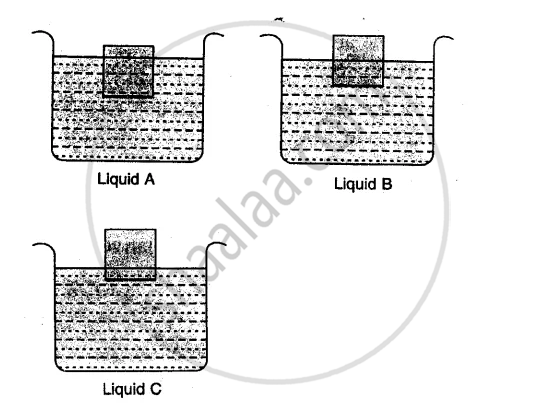ICSE Class 8CISCE
Share

# The Diagram Given Below Show a Body Floating in Three Different Liquids. A, B and C at Different Levels. (A) in Which Liquid Does the Body Experience the Greatest Buoyant Force ? (B) Which Liquid Has the Least Density ? (C) Which Liquid Has the Highest Density ? - ICSE Class 8 - Physics

ConceptConcept of Flotation and Sinking of a Substance (Relate to Density)

#### Question

The diagram given below show a body floating in three different liquids. A, B and C at different levels.
(a) In which liquid does the body experience the greatest buoyant force ?
(b) Which liquid has the least density ?
(c) Which liquid has the highest density ?#### Solution

a) Buoyant force is same in each case as the wt. of body is same in each case and Buoyant force is equal to the weight of liquid displaced by the immersed part of body which balances the wt. of body.
(b) The liquid A has the least density as body immerces the maximum.
(c) Liquid C has the highest density as the body immerces the least.

Is there an error in this question or solution?

#### APPEARS IN

Solution The Diagram Given Below Show a Body Floating in Three Different Liquids. A, B and C at Different Levels. (A) in Which Liquid Does the Body Experience the Greatest Buoyant Force ? (B) Which Liquid Has the Least Density ? (C) Which Liquid Has the Highest Density ? Concept: Concept of Flotation and Sinking of a Substance (Relate to Density).
S